Do you like the article?
Share it with others —
Find us on Telegram!
Use new possibilities of MetaTrader 5

#### Similar articles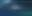# Learn how to design a trading system by Awesome Oscillator9 9634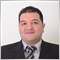### Introduction

Here is a new article in our series about learning how to design a trading system based on the most popular technical indicators for beginners through learning the root of things. We will learn a new technical tool in this article and it will be the Awesome Oscillator indicator as we will identify it in more detail to learn what it is, what it measures, and how to use it based on the main concept behind it through simple strategies, and how to create a trading system based on these mentioned strategies.

We will cover this indicator in detail the same as we mentioned through the following topics:

We will use the MQL 5 (MetaQuotes Language 5) to write our codes and the MetaTrader 5 trading terminal to execute our designed trading system. The MQL5 is built-in in the MetaTrader 5. If you want to learn how to get and use them, you can read the Writing MQL5 code in MetaEditor topic from a previous article. I advise you to download and try to apply what you learn by yourself to practice and improve your coding skills.

Disclaimer: All information provided 'as is' only for educational purposes and is not prepared for trading purposes or advice. The information does not guarantee any kind of result. If you choose to use these materials on any of your trading accounts, you will do that at your own risk and you will be the only responsible.

### Awesome Oscillator definition

In this topic, we will learn about the Awesome Oscillator (AO) indicator in more detail. AO was developed by Bill Williams to measure momentum, it can be used to clarify the power or the control with which party buyers or sellers. It measures this momentum by comparing the momentum of the last five bars or candles with the last 34 bars or candles' momentum at a bigger time frame, in other words, it compares the recent momentum with the momentum of a larger time frame. When we get a confirmation of the AO signal by another technical indicator or tool, it will be significant and more important. So, it will be better to use it accompanied by another technical tool. How we can calculate this indicator manually to understand and deepen our understanding of this indicator, if we want to learn to do that, we can do that through the following steps:

The AO is the difference between the 5- period simple moving average and the 34- period simple moving average. We will use the median price in the calculation of the AO.

MEDIAN PRICE = (HIGH + LOW) / 2

AO = SMA (MEDIAN PRICE, 5) - SMA (MEDIAN PRICE, 34)

Where:

MEDIAN PRICE = the median price of the instrument

HIGH = the highest price

LOW = the lowest price

AO = the Awesome Oscillator value

SMA = simple moving average

Nowadays, we do not need to calculate it manually as it is available in the MetaTrader 5 as a built-in among different technical indicators and all that we need is to choose it from the trading terminal to appear on the chart.

The following is how to insert it into the chart. While opening the MetaTrader 5 trading terminal, Press Insert tab --> Indicators --> Bill Williams --> Awesome Oscillator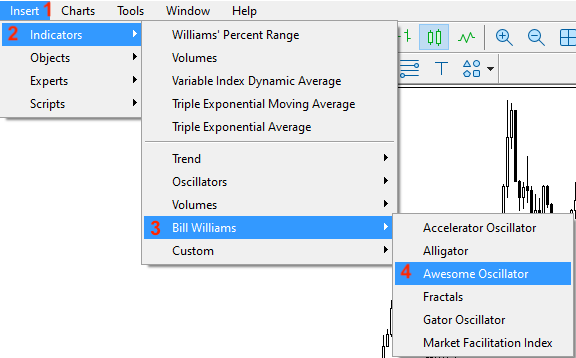After that, we can find the parameters of the AO indicator the same as the following: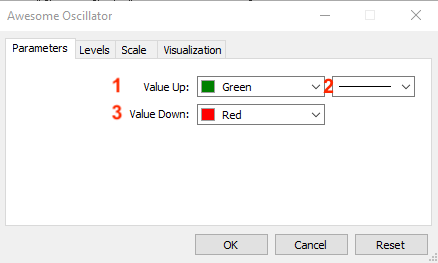Where:

1 - the color of up values

2 - the thickness of the histogram

3 - the color of down values

After determining these parameters, we will find the indicator inserted into the chart as the follows: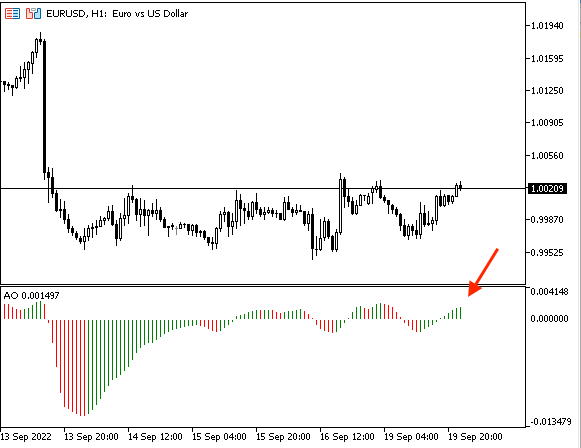As we can see in the previous chart that in the lower part of the chart we have the indicator with histogram bars oscillating around zero — this is the measurement of the momentum.

### Awesome Oscillator strategy

In this topic, we will learn how to use the AO indicator through some simple strategies for the purpose of education only based on the main concept behind the indicator. You must test any strategy of them before using them on your real account to make sure that it is useful, profitable, and suitable for your trading. Depending on that, you may find that mentioned strategies need optimization as these strategies are the same as we mentioned the main purpose of them is only for education and it is normal to find that. It will be better to use this indicator with another technical indicator to get more effective results especially if we use another technical indicator or tool that can be used to give us more insights more many different perspectives. By the way, this is one of the most important and effective features of the technical analysis as we can use many tools to look at and evaluate the underlining instrument from different perspectives and this will help us to take suitable investment or trading decisions to get good results. In this article, we will combine another technical indicator the moving average the same as we will see in this topic to get more effective buy or sell signals.

Strategy one: AO Zero Crossover

Based on this strategy we need to get a bullish or bearish signal based on the crossover between the current AO value and the zero level. If the current AO value is greater than the zero level, it will be a bullish signal. If the current AO value is lower than the zero level, it will be a bearish signal.

Simply,

The current AO > 0 --> Bullish

The current AO < 0 --> Bearish

Strategy two: AO Strength

According to this strategy, we need to get a signal of the strength of AO movement based on the position of the current AO and the average of 5- period of the last AO values. If the current AO value is greater than the AVG, it will be a signal of the strength of the AO movement. If the current AO value is lower than the AVG, it will be a signal of the weakness of the AO movement.

Simply,

The current AO > AVG 5- period of AO --> AO is strong

The current AO < AVG 5- period of AO --> AO is weak

Strategy three: AO & MA Strategy

According to this strategy, we need to get signals of buy and sell based on the position of the current AO value and the zero level in addition to the position of the closing price and 50- period exponential moving average. If the current AO value is greater than the zero level and at the same time the closing price is greater than the 50- period EMA, it will be a buy signal. If the current AO value is lower than the zero level and at the same time the closing price is lower than the EMA, it will be a sell signal.

Simply,

The current AO > the zero level and the closing price > 50- period EMA --> buy signal

The current AO < the zero level and the closing price < 50- period EMA --> sell signal

### Awesome Oscillator strategy blueprint

We will design a step-by-step blueprint for each mentioned strategy to help us to create our trading system easily by organizing our ideas.

Strategy one: AO Zero Crossover

According to this strategy, we need to create a trading system that can be able to generate signals of bullish or bearish based on continuously comparing two values and they are the current AO value and the zero level of AO indicator to determine the position of each value and decide the statue of the market. If the AO value is greater than the zero level, we need the trading system to generate a comment on the chart with the following values:

• Bullish
• AO Value is n

In the other scenario, if the current AO value is lower than the zero level, we need the trading system to return a comment on the chart with the following values:

• Bearish
• AO Value is n

The following is for the step-by-step of this trading system: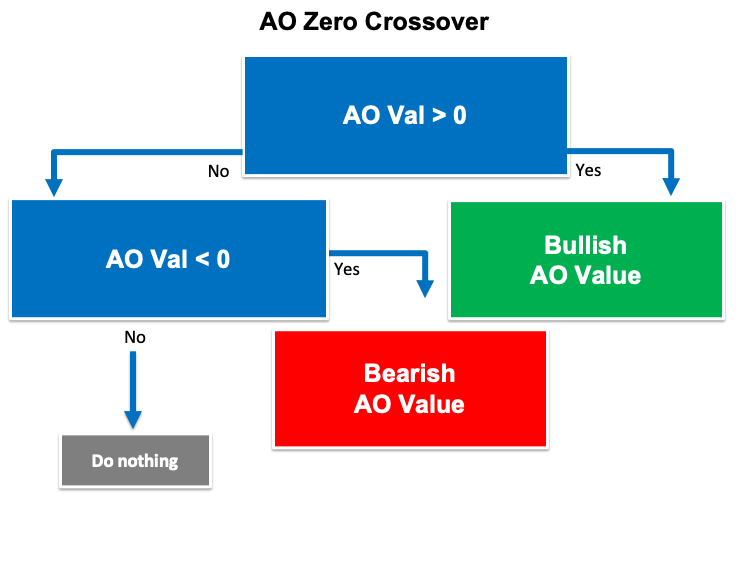Strategy two: AO Strength

According to this strategy, we need to create a trading system that can be used to generate signals of the strength of AO movement based on continuously comparing two values and which are the current AO and the average of the last five AO values before the current one to determine which one is greater. If the current AO value is greater than the AVG, we need the trading system to return a comment with the following values (Signal and values that were the reason for this signal):

• AO Movement is strong
• AO CurrVal : n
• AO FirstVal : n
• AO SecondVal : n
• AO ThirdVal : n
• AO FourthVal : n
• AO FifthVal : n
• AO AvgVal : n

In the other case, if the current AO is lower than the AVG, we need the trading system to return the following values as a comment also:

• AO Movement is Weak
• AO CurrVal : n
• AO FirstVal : n
• AO SecondVal : n
• AO ThirdVal : n
• AO FourthVal : n
• AO FifthVal : n
• AO AvgVal : n

The following is a step-by-step blueprint of this trading system: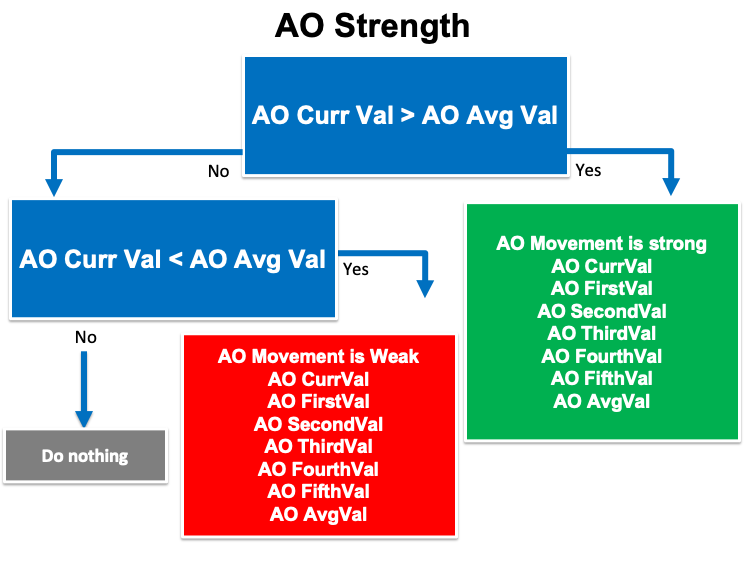Strategy three: AO & MA Strategy

Based on this strategy the same as we learned in the previous topic, we need to create a trading system that can be used to return a comment with suitable signals of buy and sell on the chart based on continuously comparing four values and they are:

• The current AO
• The zero level of the AO indicator
• The closing price
• the 50 -Period of MA

If the current AO is greater than the zero level and the closing price is greater than the 50- period MA, we need the trading system to return the following values as comment on the chart:

• Closing Price is n
• AO Value is n
• MA Value is n

In the other scenario, if the current AO value is lower than the zero level and the closing price is lower than the 50- period MA, we need the trading system to return comment on the chart with the following values:

• Sell
• Closing Price is n
• AO Value is n
• MA Value is n

The following is a step-by-step blueprint of this trading system: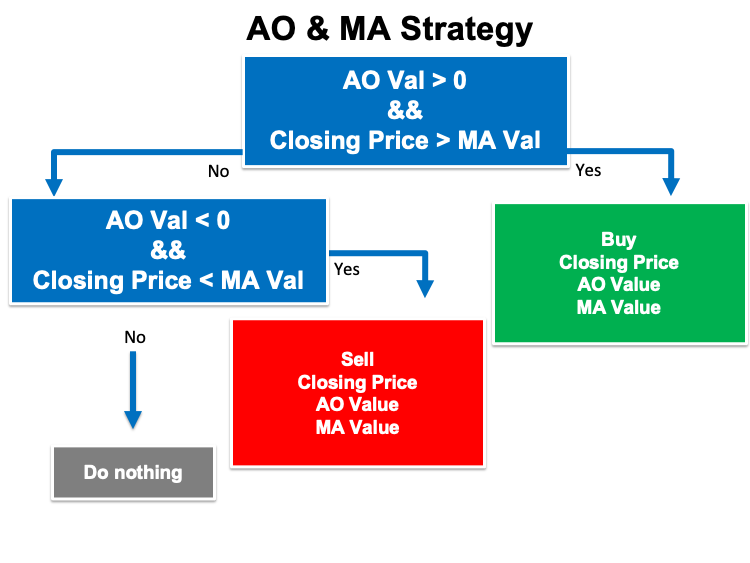In this topic, we will learn the most interesting thing in this article because we will create trading systems for our mentioned trading strategies. First, we will create a simple trading system that will generate a signal as a comment on the chart with the current AO value and we will use it as a base for other trading systems.

The following is for how to create a trading system for this desired signal step-by-step:

Creating array for the aoArray by using the "double" function:

`double aoArray[];`

Setting the AS_SERIES flag to the aoArray to return a true or false as a boolean value by using the "ArraySetAsSeries" function. Its parameters are:

• array[]: we will use aoArray.
• flag: We will use "true" as an array indexing direction.
`ArraySetAsSeries(aoArray,true);`

Defining the AO indicator by using the "iAO" function to return the handle of the Awesome Oscillator indicator. Its parameters are:

• symbol: we will use the (_Symbol) to be applied to the current symbol.
• period: we will use the (_period) to be applied to the current period.
`int aoDef = iAO(_Symbol,_Period);`

Getting data from the buffer of the AO indicator by using the "CopyBuffer" function. Its parameters are:

• indicator_handle: we will use the (aoDef) of the predefined indicator handle.
• buffer_num: to determine the indicator buffer number, we will use (0).
• start_pos: to determine the starting position, we will use (0).
• count: to determine the amount to copy, we will use (3).
• buffer[]: to determine the target array to copy, we will use (aoArray).
`CopyBuffer(aoDef,0,0,3,aoArray);`

Defining the AO value after creating a double variable for the aoVal and Normalizing it by using the "NormalizeDouble". Parameters of the "NormalizeDouble" are:

• value: Normalized number. We will use (aoArray).
• digits: to determine the number of digits after the decimal. We will use (7).
`double aoVal = NormalizeDouble(aoArray,7);`

Returning a comment on the chart with the current AO value by using the "Comment" function.

`Comment("AO Value is ",aoVal);`

So, the following is the full code to create this trading system:

```//+------------------------------------------------------------------+
//|                                                    Simple AO.mq5 |
//|                                  Copyright 2022, MetaQuotes Ltd. |
//|                                             https://www.mql5.com |
//+------------------------------------------------------------------+
#property version   "1.00"
//+------------------------------------------------------------------+
//| Expert initialization function                                   |
//+------------------------------------------------------------------+
void OnTick()
{
double aoArray[];
ArraySetAsSeries(aoArray,true);
int aoDef = iAO(_Symbol,_Period);
CopyBuffer(aoDef,0,0,3,aoArray);
double aoVal = NormalizeDouble(aoArray,7);
Comment("AO Value is ",aoVal);
}
//+------------------------------------------------------------------+```

After compiling this code and being sure that there are no errors, we will find the expert of it in the navigator window the same as the following: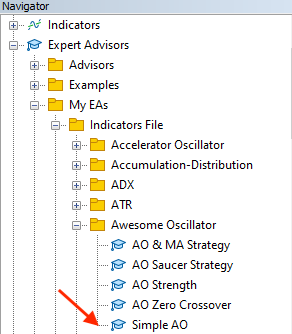By dragging and dropping this expert on the desired chart, we will see the window of this expert the same as the following: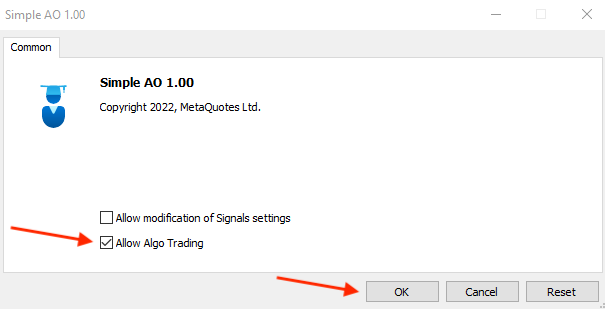After ticking next to the "Allow Algo Trading" and pressing "OK", we will find that the expert is attached to the chart the same as the following: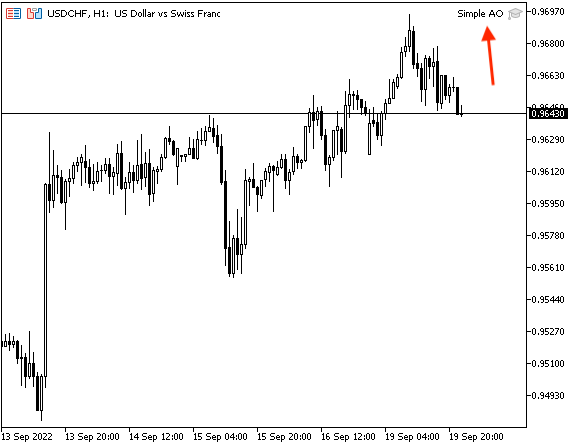As we can see on the chart in the top right corner that we have the expert is attached. Now, we're ready to receive our desired signal and it will be the same as the following: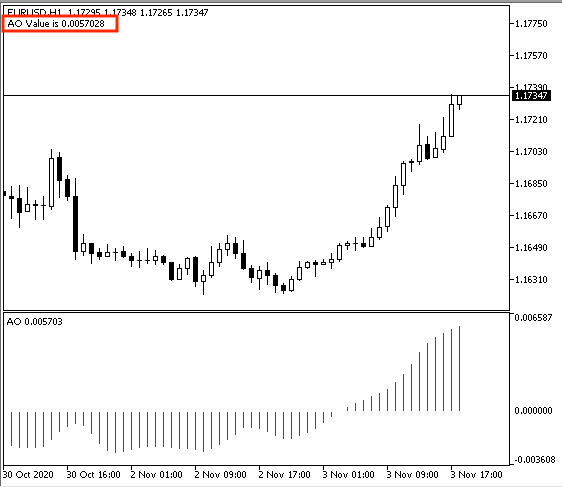As we can see on the previous chart that we have the desired signal of the current AO as a comment in the top left corner.

Strategy one: AO Zero Crossover

We will create a trading system based on this strategy to receive the desired signals and it will be the same as the following of the full code:

```//+------------------------------------------------------------------+
//|                                            AO Zero Crossover.mq5 |
//|                                  Copyright 2022, MetaQuotes Ltd. |
//|                                             https://www.mql5.com |
//+------------------------------------------------------------------+
#property version   "1.00"
//+------------------------------------------------------------------+
void OnTick()
{
double aoArray[];
ArraySetAsSeries(aoArray,true);
int aoDef = iAO(_Symbol,_Period);
CopyBuffer(aoDef,0,0,3,aoArray);
double aoVal = NormalizeDouble(aoArray,7);
if(aoVal > 0)
{
Comment("Bullish","\n"
"AO Value is ",aoVal);
}

if(aoVal < 0)
{
Comment("Bearish","\n"
"AO Value is ",aoVal);
}
}
//+------------------------------------------------------------------+```

Difference in this code.

Conditions of this strategy,

In case of bullish:

```   if(aoVal > 0)
{
Comment("Bullish","\n"
"AO Value is ",aoVal);
}```

In case of bearish:

```   if(aoVal < 0)
{
Comment("Bearish","\n"
"AO Value is ",aoVal);
}```

After compiling this code and being sure that there are no errors, we will be able to use and attach it to the chart the same what we mentioned before. So, it will be attached the same as following: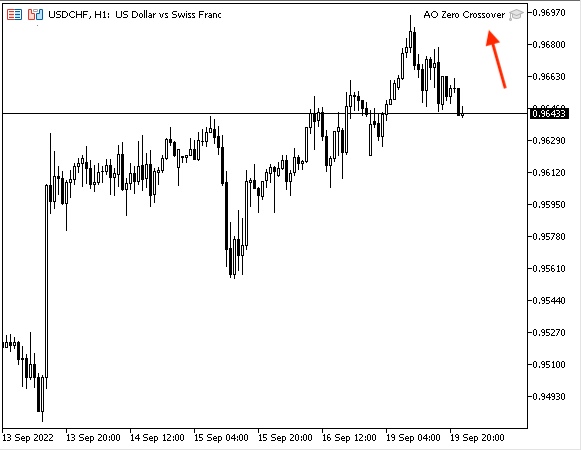As we can see on the chart that we have the expert is attached to the chart the same as it appears in the top right corner. Now, we will be ready to receive our desired signals.

In case of bullish signal: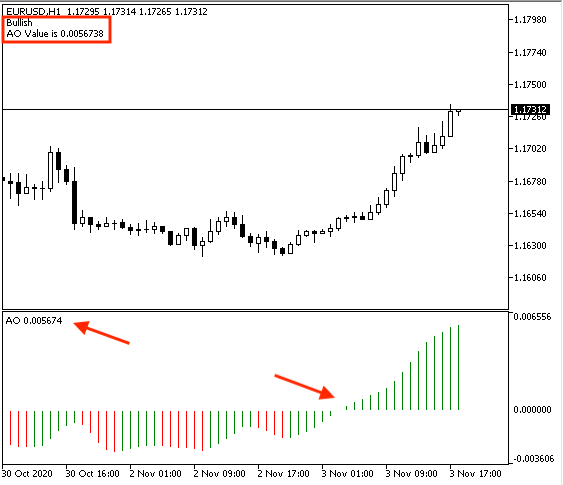As we can see in the previous chart that we have a comment in the top left corner with the following values:

• Bullish
• AO current value

In case of bearish signal: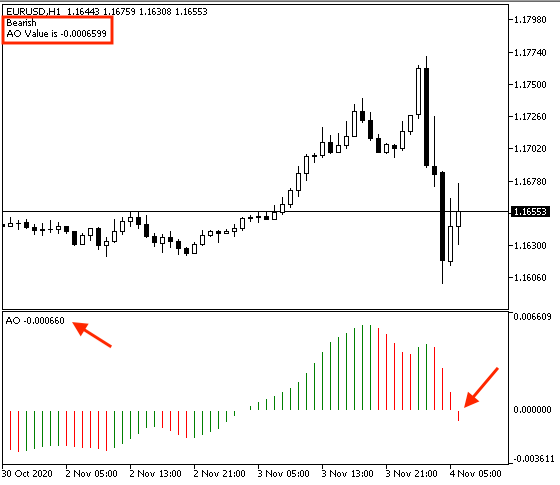As we can see on the previous chart, we have a comment as a signal with the following values:

• Bearish
• AO current value

So, we got desired signals based on this strategy, in cases of bullish and bearish.

Strategy two: AO Strength

The following is the full code of this trading system:

```//+------------------------------------------------------------------+
//|                                                  AO Strength.mq5 |
//|                                  Copyright 2022, MetaQuotes Ltd. |
//|                                             https://www.mql5.com |
//+------------------------------------------------------------------+
#property version   "1.00"
//+------------------------------------------------------------------+
void OnTick()
{
double aoArray[];
ArraySetAsSeries(aoArray,true);
int aoDef = iAO(_Symbol,_Period);
CopyBuffer(aoDef,0,0,6,aoArray);

double aoCurrVal = NormalizeDouble(aoArray,6);
double aoFifthVal = NormalizeDouble(aoArray,6);
double aoFourthVal = NormalizeDouble(aoArray,6);
double aoThirdVal = NormalizeDouble(aoArray,6);
double aoSecondVal = NormalizeDouble(aoArray,6);
double aoFirstVal = NormalizeDouble(aoArray,6);

double aoAvgVal = NormalizeDouble((aoFifthVal+aoFourthVal+aoThirdVal+aoSecondVal+aoFirstVal)/5,6);

if(aoCurrVal > aoAvgVal)
{
Comment("AO Movement is strong","\n",
"AO CurrVal : ",aoCurrVal,"\n",
"AO FirstVal : ",aoFifthVal,"\n",
"AO SecondVal : ",aoFourthVal,"\n",
"AO ThirdVal : ",aoThirdVal,"\n",
"AO FourthVal : ",aoSecondVal,"\n",
"AO FifthVal : ",aoFirstVal,"\n",
"AO AvgVal : ",aoAvgVal
);
}

if(aoCurrVal < aoAvgVal)
{
Comment("AO Movement is Weak","\n",
"AO CurrVal : ",aoCurrVal,"\n",
"AO FirstVal : ",aoFifthVal,"\n",
"AO SecondVal : ",aoFourthVal,"\n",
"AO ThirdVal : ",aoThirdVal,"\n",
"AO FourthVal : ",aoSecondVal,"\n",
"AO FifthVal : ",aoFirstVal,"\n",
"AO AvgVal : ",aoAvgVal
);
}

}
//+------------------------------------------------------------------+```

Differences in this code:

By using the "NormalizeDouble" function we will define the following values from the current value of AO back to five values:

```   double aoCurrVal = NormalizeDouble(aoArray,6);
double aoFifthVal = NormalizeDouble(aoArray,6);
double aoFourthVal = NormalizeDouble(aoArray,6);
double aoThirdVal = NormalizeDouble(aoArray,6);
double aoSecondVal = NormalizeDouble(aoArray,6);
double aoFirstVal = NormalizeDouble(aoArray,6);```

Defining the AVG of 5-period of AO:

`double aoAvgVal = NormalizeDouble((aoFifthVal+aoFourthVal+aoThirdVal+aoSecondVal+aoFirstVal)/5,6);`

Conditions of this strategy.

In case of the strength of AO movement:

```   if(aoCurrVal > aoAvgVal)
{
Comment("AO Movement is strong","\n",
"AO CurrVal : ",aoCurrVal,"\n",
"AO FirstVal : ",aoFifthVal,"\n",
"AO SecondVal : ",aoFourthVal,"\n",
"AO ThirdVal : ",aoThirdVal,"\n",
"AO FourthVal : ",aoSecondVal,"\n",
"AO FifthVal : ",aoFirstVal,"\n",
"AO AvgVal : ",aoAvgVal
);
}```

In case of the weakness of AO movement:

```   if(aoCurrVal < aoAvgVal)
{
Comment("AO Movement is Weak","\n",
"AO CurrVal : ",aoCurrVal,"\n",
"AO FirstVal : ",aoFifthVal,"\n",
"AO SecondVal : ",aoFourthVal,"\n",
"AO ThirdVal : ",aoThirdVal,"\n",
"AO FourthVal : ",aoSecondVal,"\n",
"AO FifthVal : ",aoFirstVal,"\n",
"AO AvgVal : ",aoAvgVal
);
}```

After compiling, being sure that there are no errors, and attaching the expert. We will find it the same as the following: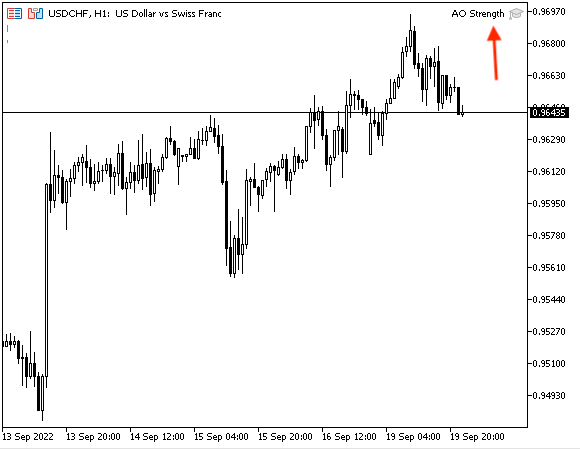As we can see on the chart in the top right corner that the expert is attached.

In case of strength: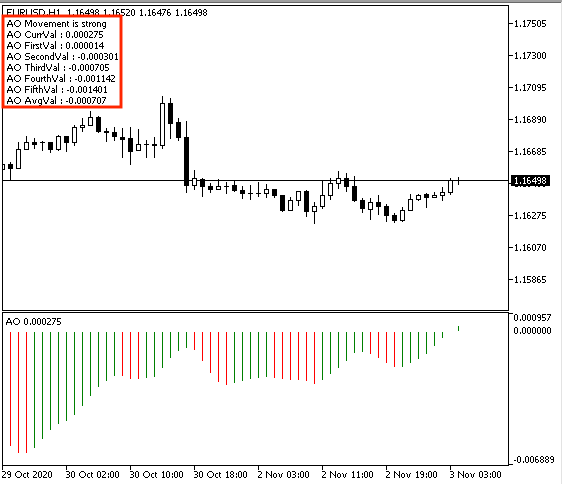As we can see on the chart in the top left corner that we have a comment with the following values:

• AO Movement is strong
• AO CurrVal : n
• AO FirstVal : n
• AO SecondVal : n
• AO ThirdVal : n
• AO FourthVal : n
• AO FifthVal : n
• AO AvgVal : n

In case of weakness: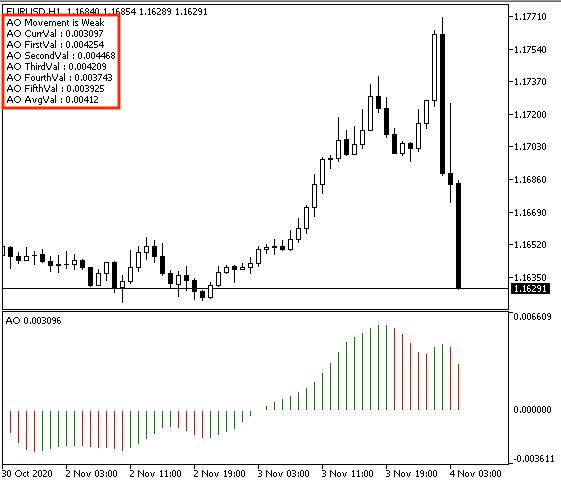As we can see on the chart in the top left corner that we have a comment with the following values:

• AO Movement is Weak
• AO CurrVal : n
• AO FirstVal : n
• AO SecondVal : n
• AO ThirdVal : n
• AO FourthVal : n
• AO FifthVal : n
• AO AvgVal : n

Now, we got desired signals based on this trading system of this strategy.

Strategy three: AO & MA Strategy

The following is the full code of this trading system.

```//+------------------------------------------------------------------+
//|                                             AO & MA Strategy.mq5 |
//|                                  Copyright 2022, MetaQuotes Ltd. |
//|                                             https://www.mql5.com |
//+------------------------------------------------------------------+
#property version   "1.00"
//+------------------------------------------------------------------+
void OnTick()
{
MqlRates pArray[];
double aoArray[];
double maArray[];

int Data=CopyRates(_Symbol,_Period,0,1,pArray);
ArraySetAsSeries(aoArray,true);
ArraySetAsSeries(maArray,true);

int aoDef = iAO(_Symbol,_Period);

CopyBuffer(aoDef,0,0,3,aoArray);

double closingPrice = pArray.close;
double aoVal = NormalizeDouble(aoArray,7);
double maVal = NormalizeDouble(maArray,7);

if(aoVal > 0 && closingPrice > maVal)
{
"Closing Price is ",closingPrice,"\n",
"AO Value is ",aoVal,"\n",
"MA Value is ",maVal);
}

if(aoVal < 0 && closingPrice < maVal)
{
Comment("Sell","\n"
"Closing Price is ",closingPrice,"\n",
"AO Value is ",aoVal,"\n",
"MA Value is ",maVal);
}

}
//+------------------------------------------------------------------+```

Differences in this code.

Creating three arrays of pArray, aoArray, and maArray. We will use the double function the same as what we mentioned except for the pArray, we will use the MqlRates function to store information about the price, volume, and spread.

```   MqlRates pArray[];
double aoArray[];
double maArray[];```

Setting the AS_SERIES flag to arrays of (aoArray) and (maArray) the same as what we mentioned before. Defining Data by using the "CopyRates" function to get historical data of MqlRates structure and its parameters are:

• symbol_name: We will use _Symbol to be applied to the current symbol.
• timeframe: We will use _Period to be applied to the current period.
• start_pos: to determine the starting position, we will use (0).
• count: to determine the data count to copy, we will use (1).
• rates_array[]: to determine the target array to copy, we will use the pArray.
```   int Data=CopyRates(_Symbol,_Period,0,1,pArray);
ArraySetAsSeries(aoArray,true);
ArraySetAsSeries(maArray,true);```

Defining the AO, MA:

AO by using the "iOA" function the same as we mentioned.

MA by using the "iMA" function, its parameters:

• symbol: we will use (_Symbol)
• period: we will use (_period)
• ma_period: to determine the period of the moving average, we will use 50
• ma_shift: to determine the horizontal shift, we will use (0)
• ma_method: to determine the type of the moving average, we will use exponential MA
• applied_price: to determine the type of useable price, we will use the closing price
```   int aoDef = iAO(_Symbol,_Period);

Getting data from the buffer of the AO and MA indicators by using the "CopyBuffer" function.

```   CopyBuffer(aoDef,0,0,3,aoArray);

Defining the closing price, aoArray, and maArray.

```   double closingPrice = pArray.close;
double aoVal = NormalizeDouble(aoArray,7);
double maVal = NormalizeDouble(maArray,7);```

Conditions of the strategy.

```   if(aoVal > 0 && closingPrice > maVal)
{
"Closing Price is ",closingPrice,"\n",
"AO Value is ",aoVal,"\n",
"MA Value is ",maVal);
}```

In case of sell signal:

```   if(aoVal < 0 && closingPrice < maVal)
{
Comment("Sell","\n"
"Closing Price is ",closingPrice,"\n",
"AO Value is ",aoVal,"\n",
"MA Value is ",maVal);
}```

After compiling this code and attaching it, we can see it attached the same as the following: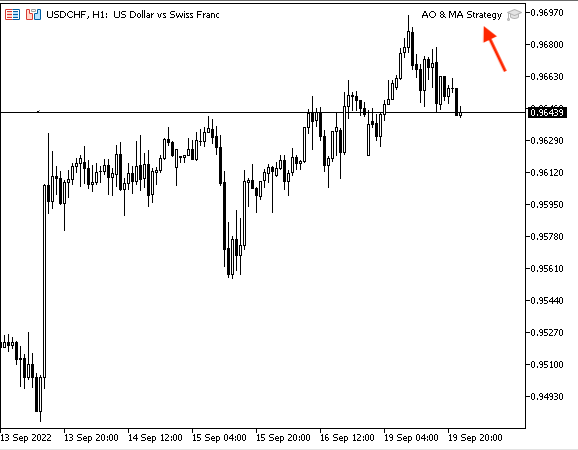As we can see that we have the indicator attached to the chart in the top left corner from the previous chart. We're ready to get our signals.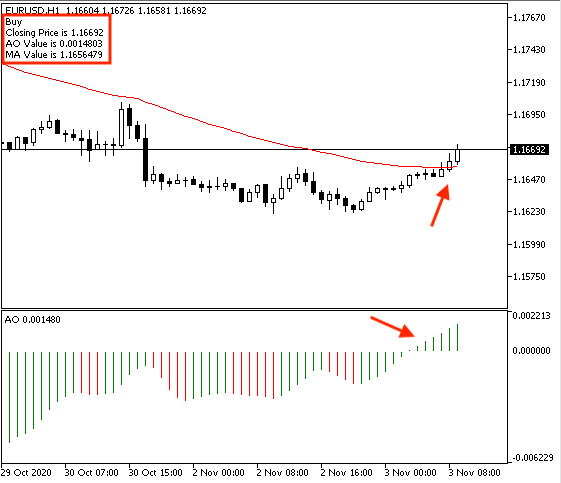As we can see that we have our signal as a comment in the top left corner with the following values:

• Closing Price is n
• AO Value is n
• MA Value is n

In case of sell: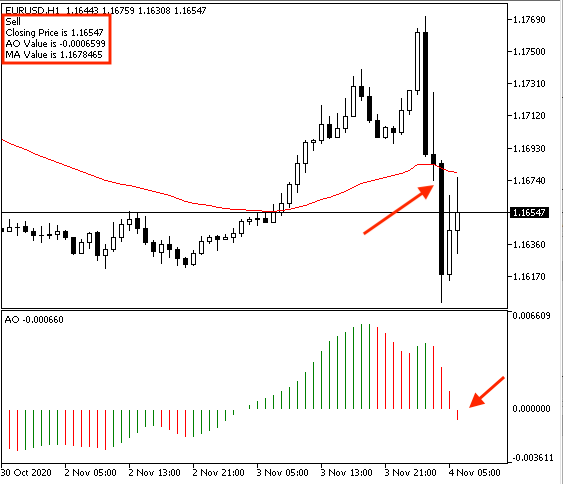We can see the comment in this case with the following values of our signal:

• Sell
• Closing Price is n
• AO Value is n
• MA Value is n

### Conclusion

Now, it is supposed that you understood the Awesome Oscillator indicator in detail as you learned what is the AO, what it measures, how we can calculate it manually, and how we can insert it into the chart from the built-in indicators of the MetaTrader 5. We learned also how we could use it through simple strategies based on the main concept behind it after understanding it, these strategies were:

• Strategy one: AO Zero Crossover - to get signals of bullish or bearish based on the zero crossovers.
• Strategy two: AO Strength - to get a signal of the strength of the AO movement based on the position of the current AO value and the AVG of the last 5- AO values.
• Strategy three: AO & MA Strategy - to get signals of buy or sell based on the position of the current AO and the zero level and the position of the closing price and the 50-period EMA.

After that, we designed a step-by-step blueprint for each mentioned strategy to create a trading system easily, smoothly, and effectively after organizing our ideas. Then we created a trading system for each mentioned strategy to get automation signals after executing them in the MetaTrader 5 trading terminal.

I hope that you tried to apply what you learned by yourself as this is a very important step in any learning process if we need to develop and improve our skills in addition that this will give you more insights about what you learn or about any related topics. I need to confirm here again that you must test any mentioned strategy before using it to make sure that it is suitable for your trading as there is nothing suitable for everyone.

Attached files |
Simple_AO.mq5 (1.01 KB)
AO_Strength.mq5 (2.07 KB)

#### Other articles by this author

Last comments | Go to discussion (4)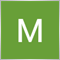| 21 Sep 2022 at 17:27
MetaQuotes:

New article Learn how to design a trading system by Awesome Oscillator has been published:

Author: Mohamed Abdelmaaboud

Thank you for your Great work, please make one based on Williams Fractals.| 28 Sep 2022 at 20:37
MANESA MANESA #:
Thank you for your Great work, please make one based on Williams Fractals.

You're welcome, I will try.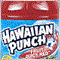| 4 Nov 2022 at 19:21
Thank you @Mohamed Abdelmaaboud for these wonderful articles.  Unfortunately, I have an account with Oanda and they do not allow MT5 because I am in Europe (Ireland) they said.  I was wondering if you would be so kind as to create this article for MQL4?  As I am just learning to code some EAs, I think this one would be a great starting point.  I tried to put some of this code into MQL4 and got some compile errors. I have written some EAs myself but they have not been successful on demo accounts. How do you normally test these EAs?  Is it through Metatrader Test suite itself or do you use a separate testing software package like Forex Tester 5?  I would like to understand how to properly test an EA and see how well it works in different market conditions.  It would be great to know that the EA works well in some market conditions before trying it out on a demo etc.  Perhaps you could create a separate article on how this can be achieved.  Thanks again for your contributions here.| 5 Nov 2022 at 08:30
Brian Tansey #:
Thank you @Mohamed Abdelmaaboud for these wonderful articles.  Unfortunately, I have an account with Oanda and they do not allow MT5 because I am in Europe (Ireland) they said.  I was wondering if you would be so kind as to create this article for MQL4?  As I am just learning to code some EAs, I think this one would be a great starting point.  I tried to put some of this code into MQL4 and got some compile errors. I have written some EAs myself but they have not been successful on demo accounts. How do you normally test these EAs?  Is it through Metatrader Test suite itself or do you use a separate testing software package like Forex Tester 5?  I would like to understand how to properly test an EA and see how well it works in different market conditions.  It would be great to know that the EA works well in some market conditions before trying it out on a demo etc.  Perhaps you could create a separate article on how this can be achieved.  Thanks again for your contributions here.

Thanks a lot Brian Tansey for your kind comment.

Unfortunately, I cannot provide here new articles by MQL4 as they are not available or not supported now. You can test any coded strategy by the strategy tester of MetaTrader as I do. You can find it in the View tab of MetaTrader --> Strategy Tester or by pressing Ctrl+R while opening the MetaTrader.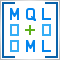Matrix and Vector operations in MQL5
Matrices and vectors have been introduced in MQL5 for efficient operations with mathematical solutions. The new types offer built-in methods for creating concise and understandable code that is close to mathematical notation. Arrays provide extensive capabilities, but there are many cases in which matrices are much more efficient.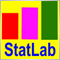The price movement model and its main provisions (Part 2): Probabilistic price field evolution equation and the occurrence of the observed random walk
The article considers the probabilistic price field evolution equation and the upcoming price spike criterion. It also reveals the essence of price values on charts and the mechanism for the occurrence of a random walk of these values.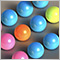MQL5 Wizard techniques you should know (Part 03): Shannon's Entropy
Todays trader is a philomath who is almost always looking up new ideas, trying them out, choosing to modify them or discard them; an exploratory process that should cost a fair amount of diligence. These series of articles will proposition that the MQL5 wizard should be a mainstay for traders.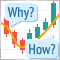Learn how to design a trading system by Relative Vigor Index
A new article in our series about how to design a trading system by the most popular technical indicator. In this article, we will learn how to do that by the Relative Vigor Index indicator.